# Vectors: Lines

Vectors: Lines

A chance to apply mathematics to 2D and 3D contexts.

Syllabus content: AHL 3.16, AHL 3.17, AHL 3.18

I have made 8 worksheets and resources to help with this topic.  They are:

1. Vector basics
2. Vector basics dot product
3. Vectors equations of lines
4. Vectors intersection of lines
5. Vectors Cartesian and cross product
6. Treasure hunt:  Vector dot product
7. Treasure hunt:  Vector equations
8. Vectors: Use of Geogebra

You can scroll down below to see the pictures questions.  You can download both the questions and mark scheme from the bottom of the page.

1. Vector basics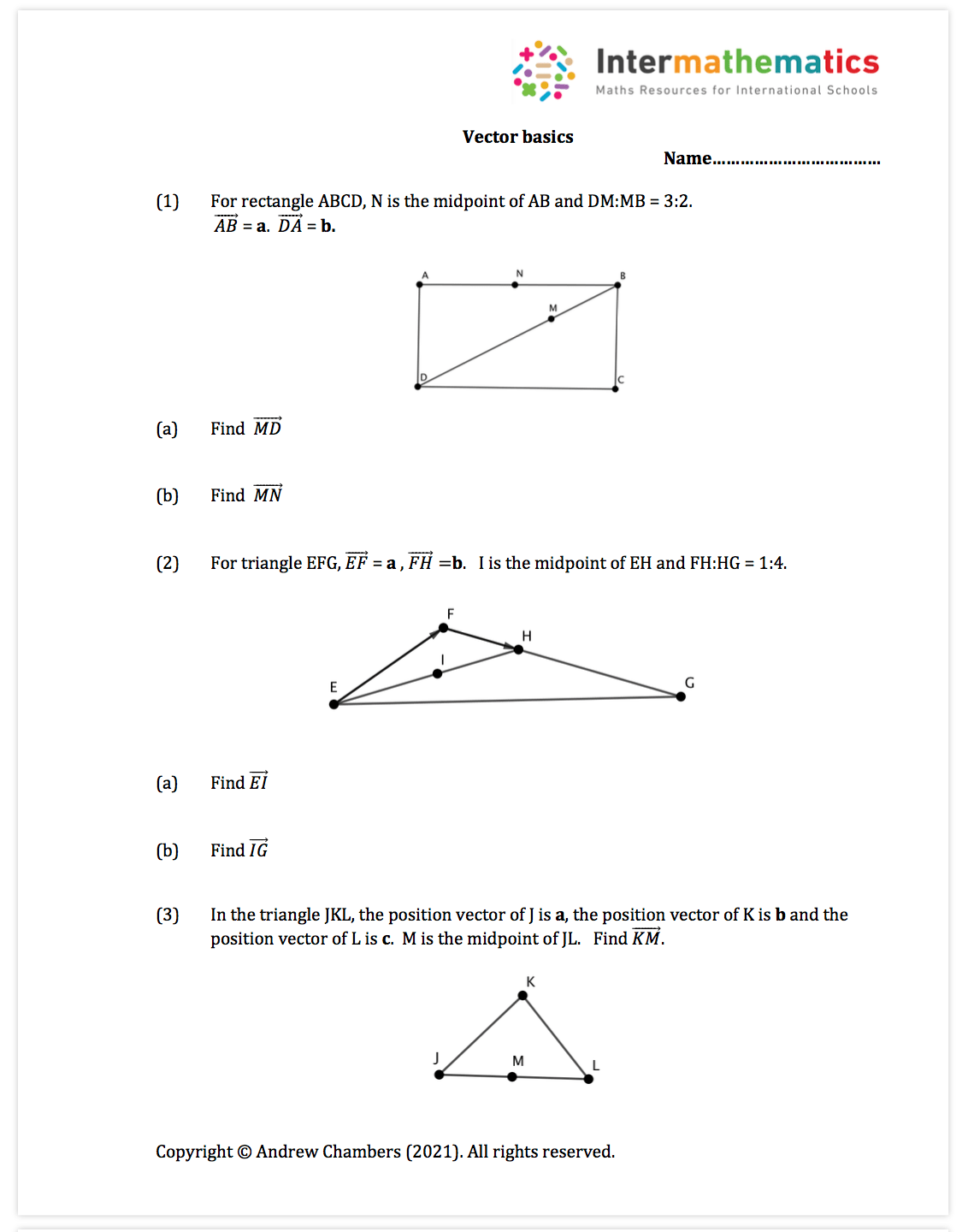Note:  This is a short worksheet which can be used in lesson or for homework.  It covers syllabus point: AHL 3.12 which looks at the basics of vector geometry.

2. Vector basics dot productNote:  This is a short worksheet which can be used in lesson or for homework.  It covers syllabus point: AHL 3.13 which looks at the use of the dot product and other basic vector properties.

3. Vectors equations of lines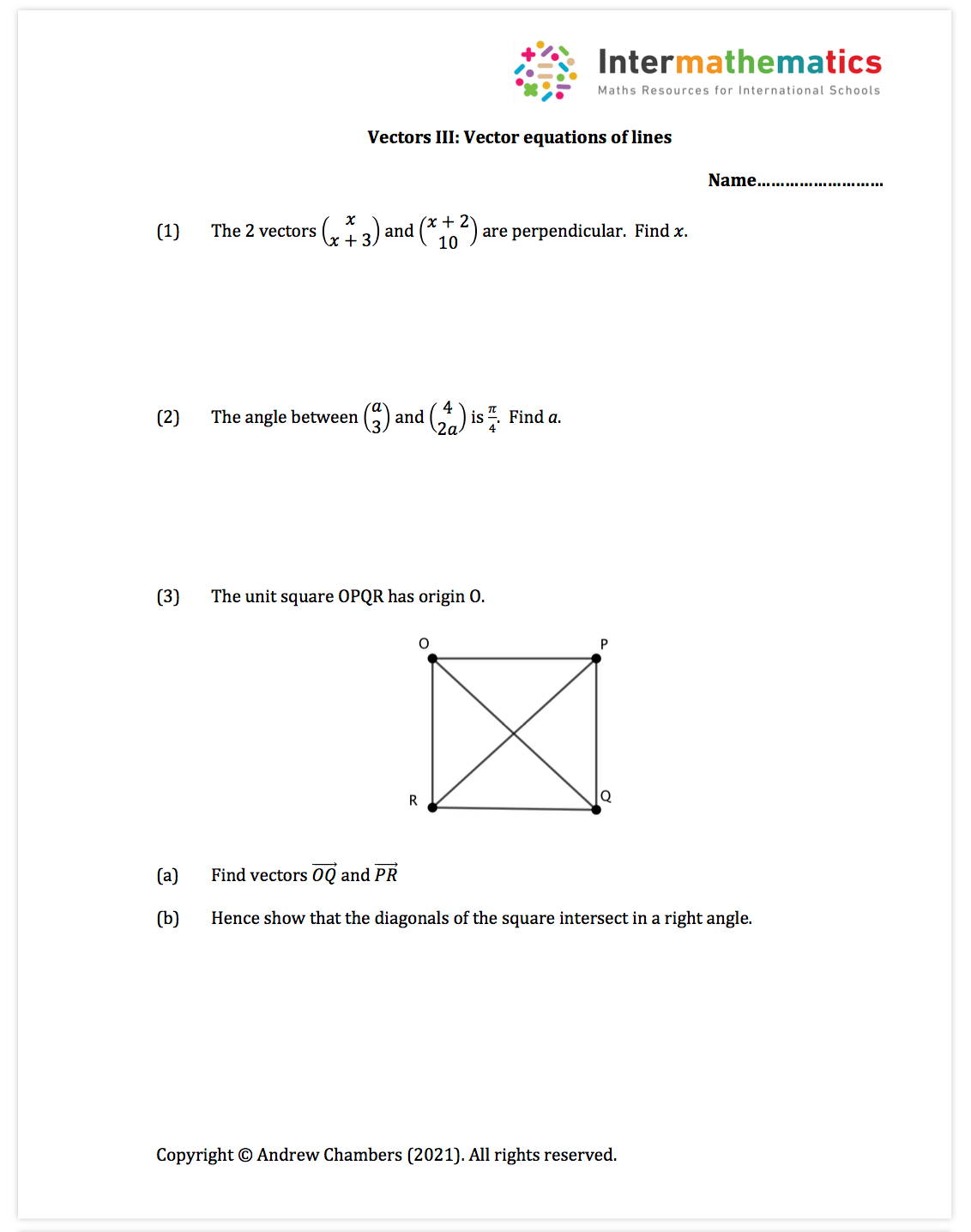Note:  This is a short worksheet which can be used in lesson or for homework.  It covers syllabus point: AHL 3.14 which looks at the vector equation of lines and angles between lines.

4. Vectors intersection of lines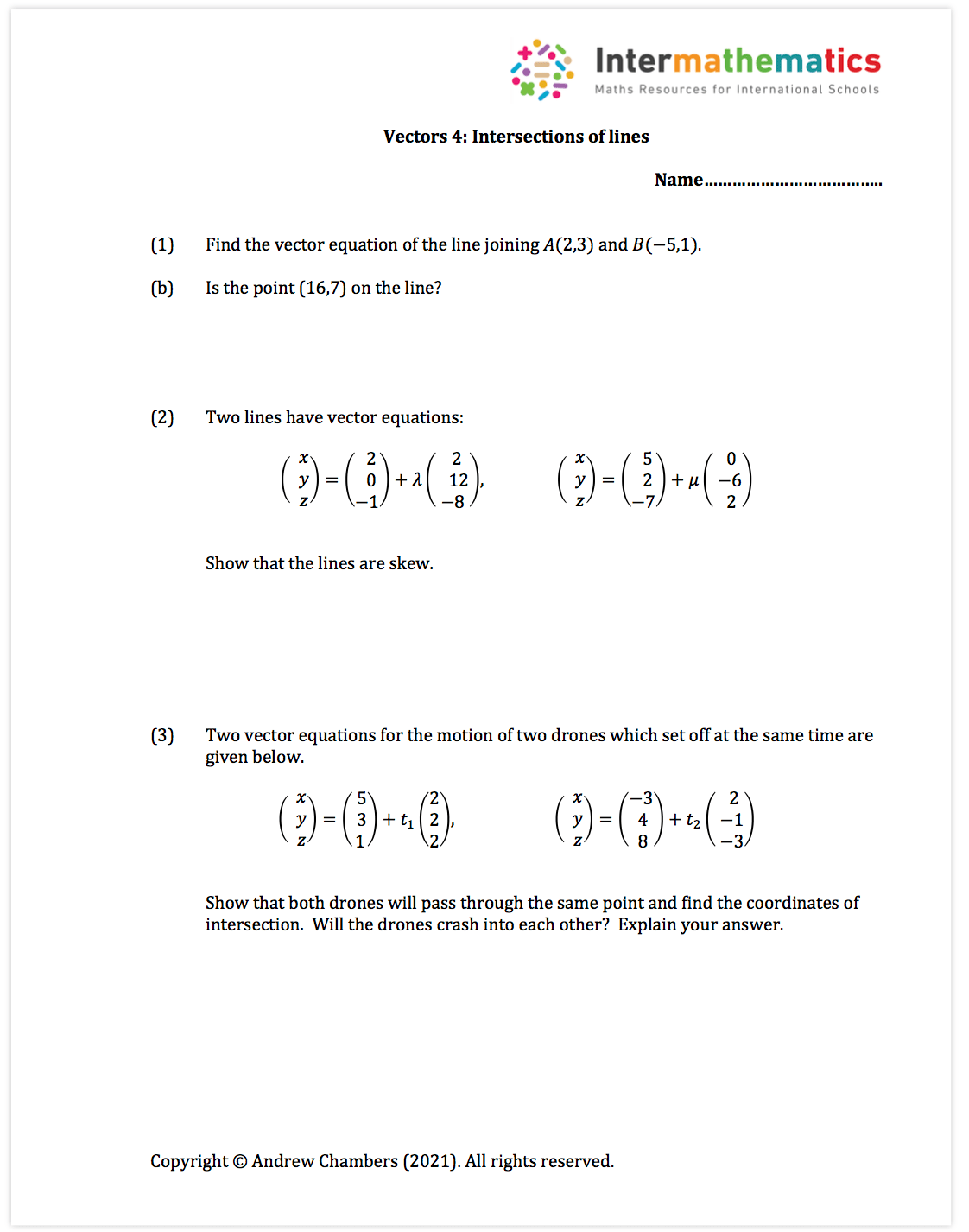Note:  This is a short worksheet which can be used in lesson or for homework.  It covers syllabus point: AHL 3.14 which looks at vector equations of lines.

5. Vectors Cartesian and cross productNote:  This is a short worksheet which can be used in lesson or for homework.  It covers syllabus point: AHL 3.15 which looks at the intersection of lines in 2D and 3D.

Treasure hunt:  Vector dot productNote:  This is an activity which can be done in pairs or as a whole class activity.  Students solve a mixture of problems relating to the dot product and angles between vectors.

Treasure hunt:  Vector equations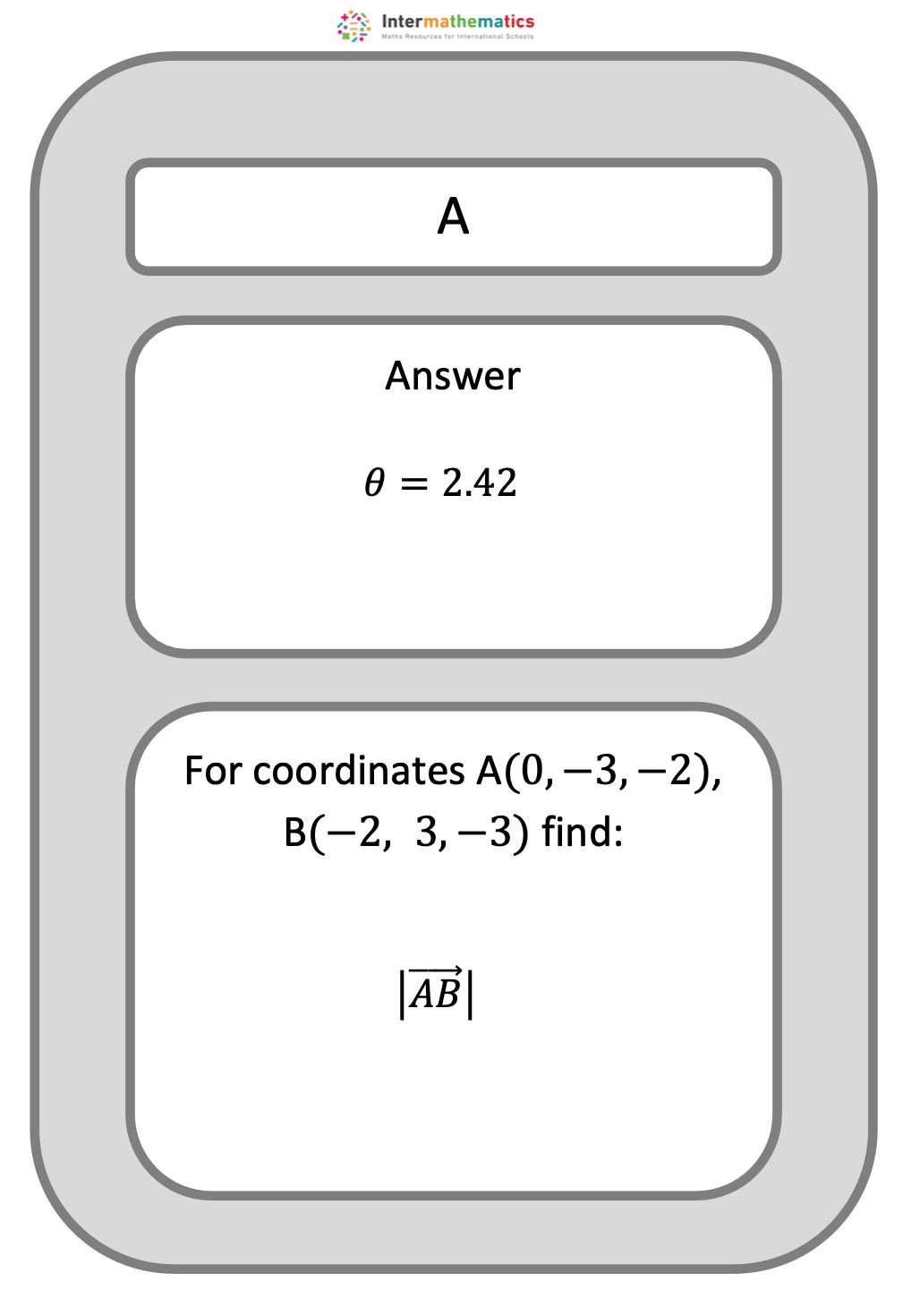Note:  This is an activity which can be done in pairs or as a whole class activity.  Students solve a mixture of vector equation problems including angles, intersections, unit vectors and distances between points.

Vectors:  Neural Networks investigationNote:  This is an investigation into the use of vectors in neural networks and artificial intelligence.

Vectors: Use of Geogebra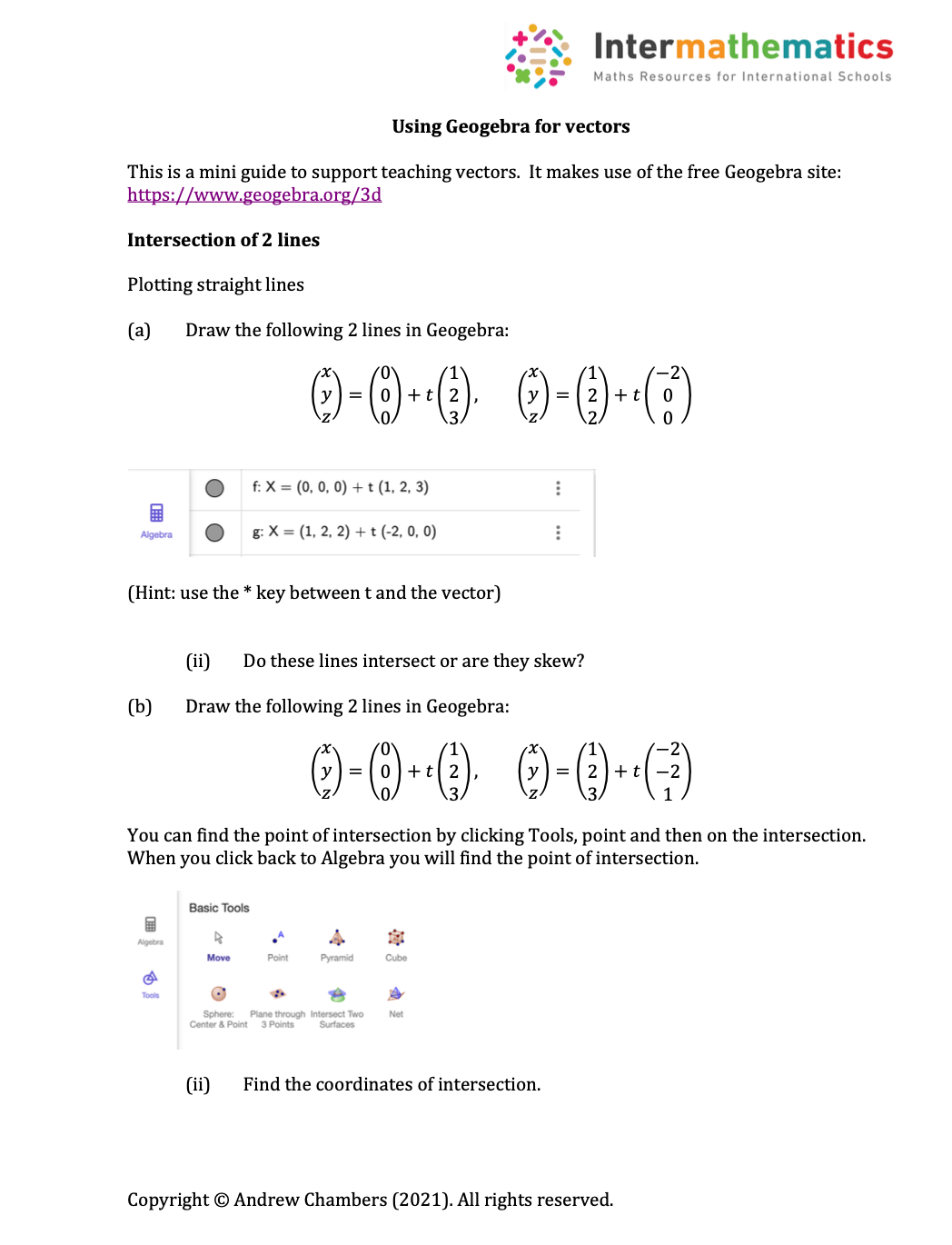Note: This is a guide I’ve made to support students in getting a good visual understanding of vectors through the online Geogebra site.  Students learn how to find intersections and angles of lines and extend into plane problems.  This would also support an interesting IA.